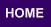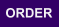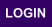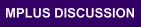Mplus
Sunday
October 01, 2023Mplus Mplus at a GlanceGeneral DescriptionMplus Programs Pricing Version History System Requirements Platforms
 Analyses/ResearchMplus Examples Papers References
 Search

Chapter 12: Monte Carlo Simulation Studies

12.1: Monte Carlo simulation study for a CFA with covariates (MIMIC) with continuous factor indicators and patterns of missing data ex12.1 ex12.1.inp none
12.2: Monte Carlo simulation study for a linear growth model for a continuous outcome with missing data where attrition is predicted by time-invariant covariates (MAR) ex12.2 ex12.2.inp none
12.3: Monte Carlo simulation study for a growth mixture model with two classes and a misspecified model ex12.3 ex12.3.inp none
12.4: Monte Carlo simulation study for a two-level growth model for a continuous outcome (three-level analysis) ex12.4 ex12.4.inp none
12.5: Monte Carlo simulation study for an exploratory factor analysis with continuous factor indicators ex12.5 ex12.5.inp none
12.6 Step 1: Monte Carlo simulation study where clustered data for a two-level growth model for a continuous outcome (three-level analysis) are generated, analyzed, and saved ex12.6step1 ex12.6step1.inp none
12.6 Step 2: External Monte Carlo analysis of clustered data generated for a two-level growth model for a continuous outcome using TYPE=COMPLEX for a single-level growth model ex12.6step2 ex12.6step2.inp ex12.6replist.dat
12.7 Step 1: Real data analysis of a CFA with covariates (MIMIC) for continuous factor indicators where the parameter estimates are saved for use in a Monte Carlo simulation study ex12.7step1 ex12.7step1.inp ex12.7real.dat
12.7 Step 2: Monte Carlo simulation study where parameter estimates saved from a real data analysis are used for population parameter values for data generation and coverage ex12.7step2 ex12.7step2.inp ex12.7estimates.dat
12.8: Monte Carlo simulation study for discrete-time survival analysis ex12.8 ex12.8.inp none
12.9: Monte Carlo simulation study for a two-part (semicontinuous) growth model for a continuous outcome ex12.9 ex12.9.inp none
12.10: Monte Carlo simulation study for a two-level continuous-time survival analysis using Cox regression with a random intercept and a frailty ex12.10 ex12.10.inp none
12.11: Monte Carlo simulation study for a two-level mediation model with random slopes ex12.11 ex12.11.inp none
12:12 Monte Carlo simulation study for a multiple group EFA with continuous factor indicators with measurement invariance of intercepts and factor loadings ex12.12 ex12.12.inp none